#### Daughter Sets

We need to represent labeled edges between nodes. Traditionally, this is realized by means of feature structures: each node is a feature structure and an edgeis represented by the presence of a featureon, such that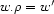. This, however, is a lousy idea when the goal is to take advantage of active constraint propagation. The problem is that, in the traditional view, features are partial functions: i.e.is a partial function from nodes to nodes (from now on, we will write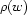instead of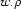). It is rather inconvenient to try to express constraints onwhen it is not always defined!

However, a slight change of representation allows us to turninto a total function. Instead ofbeing either undefined or defined and denoting a node, we let it denote a set of nodes. Now instead of being undefinedis simply empty. In the case where it was originally defined, it now denotes a singleton set.

Thusdenotes the set of subjects of: empty except whenis a finite verb, in which case it is a singleton. We say thatis a daughter set of, i.e. a set of daughters. This idea has the second advantage that, in addition to complements (like subject), it also naturally accommodates modifiers (like adjectives):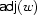is the set of adjectives of. The difference is that a modifier daughter set may have any number of elements instead of at most 1 for a complement daughter set.

Formally, for each role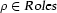, we introduce a functionsuch thatis the set of immediate daughters ofwhose dependency edge is labeled with: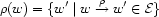in the constraint modelis a finite set variable.

Denys Duchier
Version 1.2.0 (20010221)